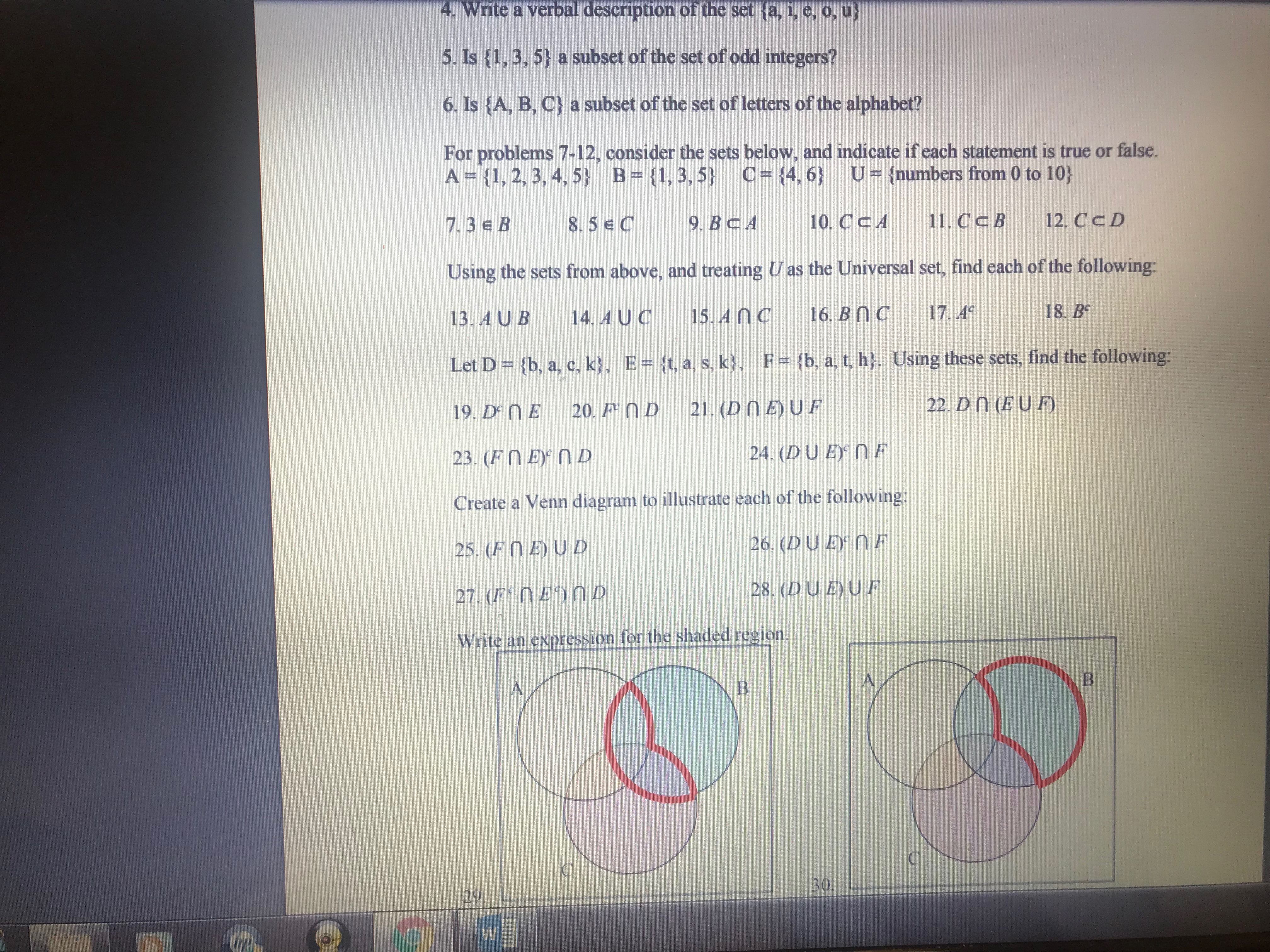# 4. Write a verbal description of the set ta, i, e, o, u 5. Is (1,3, 5) a subset of the set of odd integers? 6. 1s {A, B, C} a subset of the set of letters of the alphabet? For problems 7-12, consider the sets below, and indicate if each statement is true or false. A 11, 2, 3, 4,5) B ,3,5 C14,6) Ufnumbers from 0 to 10) 7.3e B 8.59. BcA10. CCA 1. CcB 12. CcD Using the sets from above, and treating U as the Universal set, find each of the following: 13. A UB 14. AUC 15.AnC 16.BnC 17.e 18. Be Let D- (b, a, c, k, E- It, a, s, k), F (b, a, t, h). Using these sets, find the following: 19. D NE 20. D 21. (DnE)UF 23. (FnE n D Create a Venn diagram to illustrate each of the following: 25. (F EJUD 22. Dn (EUF) 24. (DUE NF 26. (DUE)NF 28. (DUEUF Write an expression for the shaded region. 30 29

Question

20 and 22help_outlineImage Transcriptionclose4. Write a verbal description of the set ta, i, e, o, u 5. Is (1,3, 5) a subset of the set of odd integers? 6. 1s {A, B, C} a subset of the set of letters of the alphabet? For problems 7-12, consider the sets below, and indicate if each statement is true or false. A 11, 2, 3, 4,5) B ,3,5 C14,6) Ufnumbers from 0 to 10) 7.3e B 8.59. BcA10. CCA 1. CcB 12. CcD Using the sets from above, and treating U as the Universal set, find each of the following: 13. A UB 14. AUC 15.AnC 16.BnC 17.e 18. Be Let D- (b, a, c, k, E- It, a, s, k), F (b, a, t, h). Using these sets, find the following: 19. D NE 20. D 21. (DnE)UF 23. (FnE n D Create a Venn diagram to illustrate each of the following: 25. (F EJUD 22. Dn (EUF) 24. (DUE NF 26. (DUE)NF 28. (DUEUF Write an expression for the shaded region. 30 29 fullscreen

### Want to see this answer and more?

Experts are waiting 24/7 to provide step-by-step solutions in as fast as 30 minutes!*

*Response times may vary by subject and question complexity. Median response time is 34 minutes for paid subscribers and may be longer for promotional offers.
Tagged in
Math
Algebra

### Other